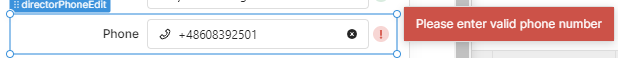# Regex validator not working for text input

Hello, I am using Regex validation in phone number input element. Here is my regex for the same : `/^\+?\d{3}?\d{3}?\d{3,11}\$/`
When I am running this regex in Retool regex tester, It is working fine, but in text input validation, it's not working well. You can see in attachment, number is fine but, it is giving error for correct numbers.﻿`^\s*(?:\+?(\d{1,3}))?[-. (]*(\d{3})[-. )]*(\d{3})[-. ]*(\d{4})(?: *x(\d+))?\s*\$`